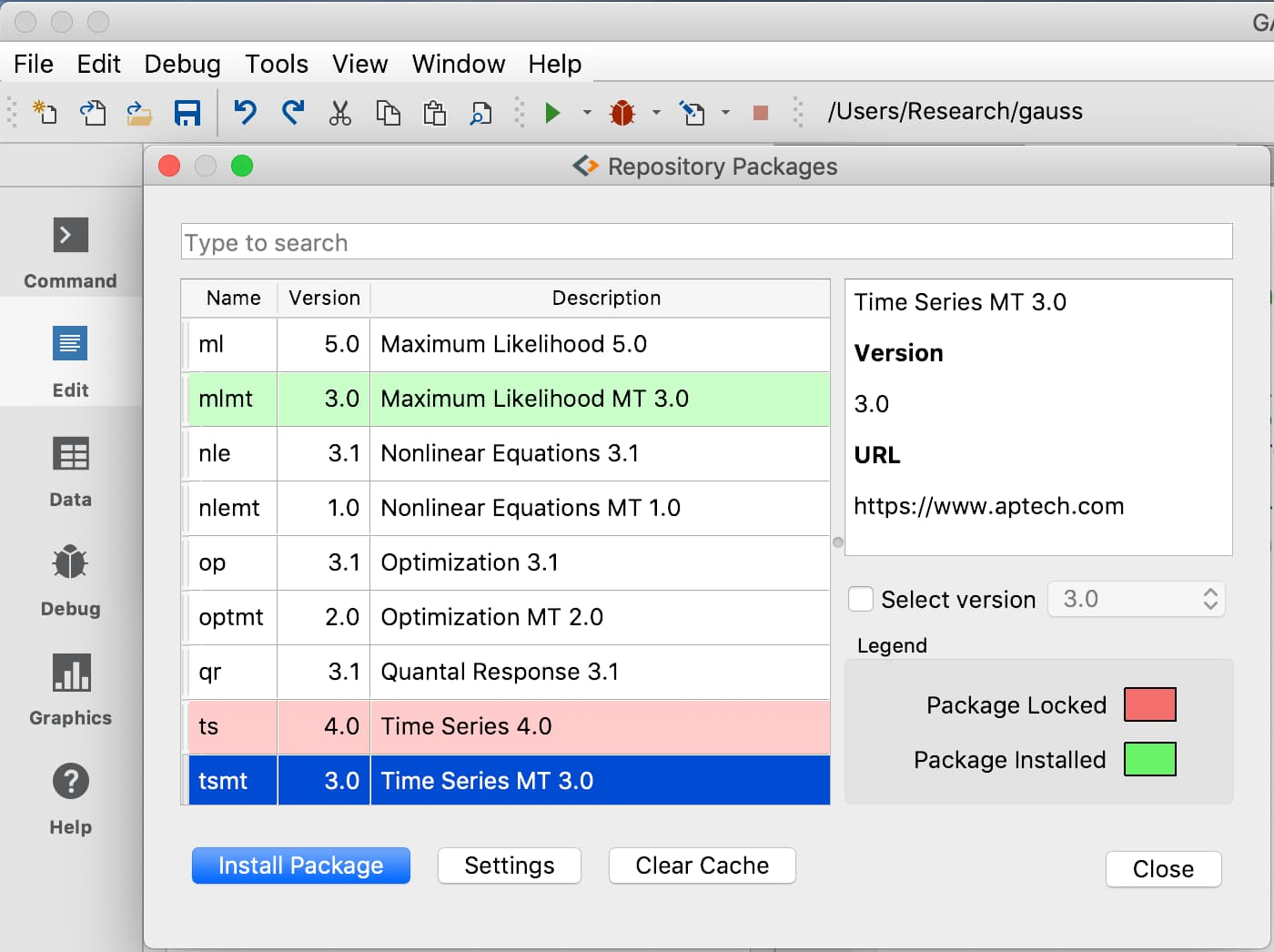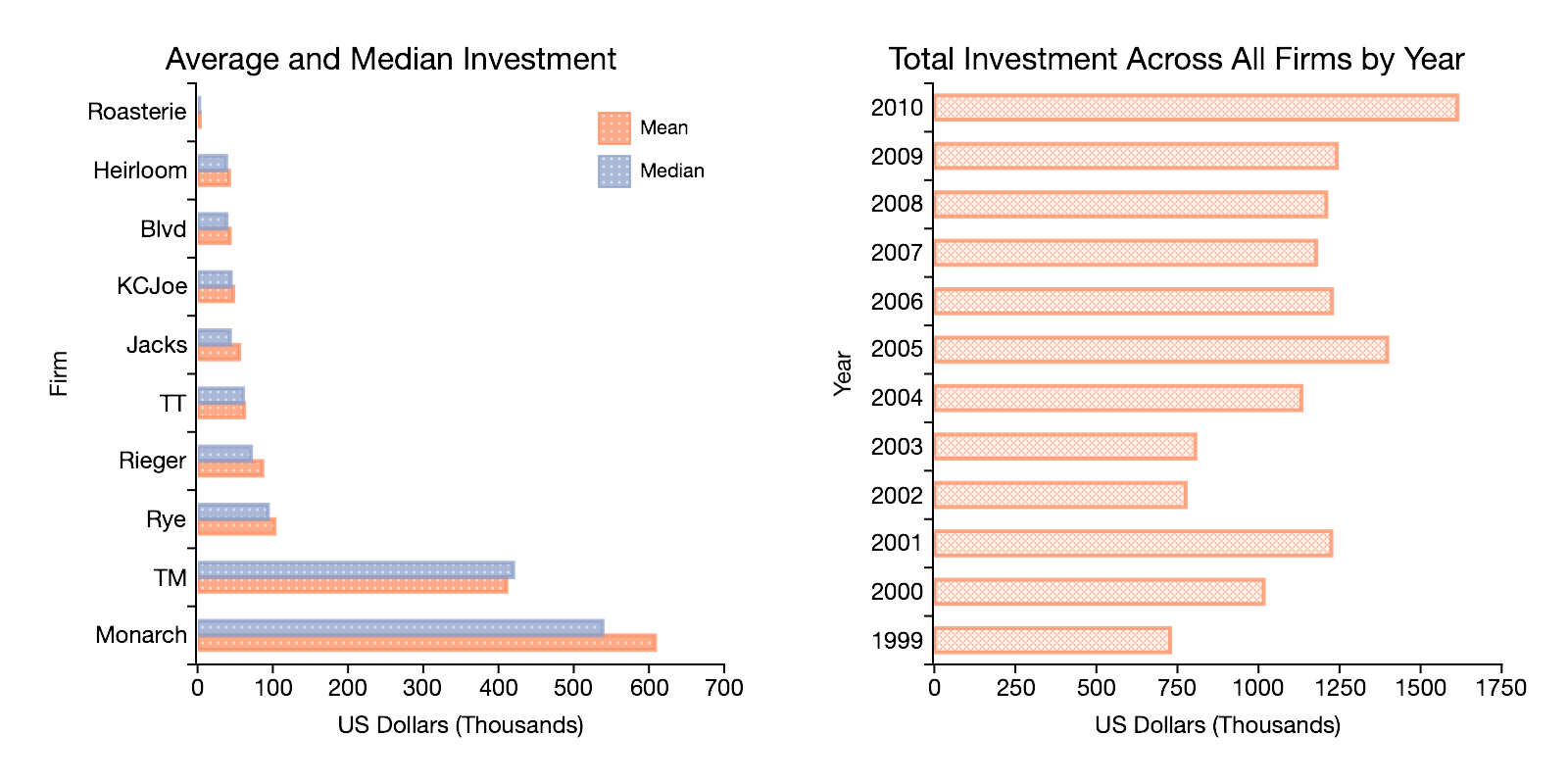# What's new in GAUSS 20

• Supports paid GAUSS Application Modules and new free GAUSS packages!## Improved panel data functionality

New function to aggregate results across groups by:

• mean, min, max, median, mode, variance, sum and standard deviation.### Example aggregate by year

{prism lang="javascript" plugins="line-numbers"} // Load data with the grouping data in the first column X = loadd("grunfeld.dat", "Years + Investment"); // Group investment by average for year mean_by_year = aggregate(X, "mean"); {/prism}

### Example aggregate by firm

{prism lang="javascript" plugins="line-numbers"} // Load data with the grouping data in the first column X = loadd("grunfeld.dat", "firm + Investment"); // Group investment by median for each firm median_by_firm = aggregate(X, "median"); {/prism}

## Advanced imputation methods for missing values

• New support for predictive mean matching, local residual draws, and linear prediction imputation.
• Customizable with options for the number of donors, matching type, and linear prediction methods.

New suite of tools makes it easy to add optional arguments to your GAUSS procedures.

• Easily integrate default values.
• Retrieve single or multiple optional inputs in a single line.
• Tools to count the number of optional inputs and check input types.

### Example estimation procedure with optional lambda

This example shows a simple estimation procedure that chooses either OLS estimation or ridge regression based on whether an optional input, lambda, is passed in.

{prism lang="javascript" plugins="line-numbers"} // ... is a placeholder for the optional arguments proc (1) = estimate(y, X, ...); local lambda; // Get the optional 'dynamic argument' lambda = getDynargs(1); // If the 'lambda' was not passed in, // it will be an empty matrix. if isempty(lambda); // No 'lambda' so perform standard OLS b_hat = olsRegress(y, X); else; // 'lambda' passed in so perform ridge regression b_hat = ridgeRegress(y, X, lambda); endif; retp(b_hat); endp; {/prism}

The procedure above can be called like this:

{prism lang="javascript" plugins="line-numbers"} // OLS regression when optional input is not passed in b_hat = estimate(y, X); {/prism}

or like this:

{prism lang="javascript" plugins="line-numbers"} // Ridge regression is performed when optional input is passed in b_hat = estimate(y, X, lambda); {/prism}

## Expanded graphics tools

### Other new graphics functionality

• `plotSetLegend` now supports setting the legend location by coordinates.
• Precise control over y-axis tick location and intervals using `plotSetYTicInterval`.
• Alpha channel support provides optional transparency for any graph element.
• Better control over bar plot adds.
• Control for legend border properties.

## Other new functions

• `modec` - Compute mode for each matrix column.
• `loaddsa` - Load string data from CSV, Excel, GAUSS, SAS or STATA datasets.
• `sprintf` - Create formatted string output from columns of matrices and strings. {prism lang="javascript" plugins="line-numbers"} var_names = "alpha" \$| "beta" \$| "gamma"; b = { 0.34, 1.9334, -0.8983 }; se = { 0.00002234, 0.013235, 0.03752 }; {/prism} {prism lang="javascript" plugins="line-numbers"} print sprintf(fmt, var_names, b, se); {/prism} {prism lang="javascript" plugins="line-numbers"} alpha 0.340 (0.00) beta 1.933 (0.01) gamma -0.898 (0.04) {/prism}
• `weighted ols` - Compute weighted OLS estimates with user-specified weights.